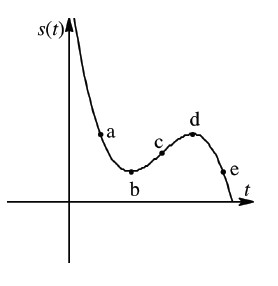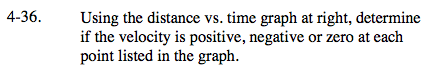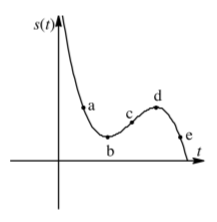### Home > CALC > Chapter 4 > Lesson 4.1.3 > Problem4-36

4-36.
1. Using the distance vs. time graph below, determine if the velocity is positive, negative or zero at each point listed in the graph. Homework Help ✎Since the slope at a point is the same as the slope of the tangent line at that point,draw small tangent lines. Use their slopes to answer these questions.